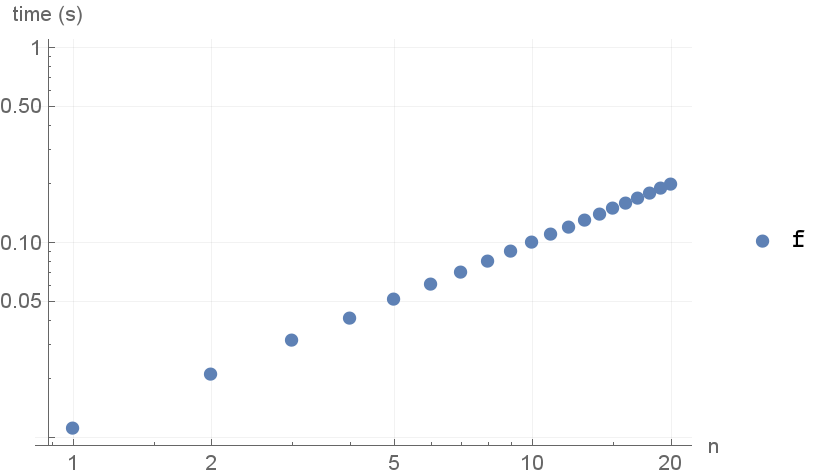# EvaluateBenchmark

Contributed by: Taliesin Beynon

Measure the evaluation timings of a function on a given set of inputs

 ResourceFunction["EvaluateBenchmark"][f,g,{n1,n2,…}] gives {{n1,t1},{n2,t2},…}, where ti is the time taken to evaluate f on the input g[ni], as measured by RepeatedTiming. ResourceFunction["EvaluateBenchmark"][f,g] chooses the ni adaptively by observing the execution of f.

## Details and Options

ResourceFunction["EvaluateBenchmark"] effectively uses Monitor.
ResourceFunction["EvaluateBenchmark"] takes the following options:
 TimeConstraint 10 maximum time to run tests

## Examples

### Basic Examples

Get the timings for Inverse of random matrices:

 In:=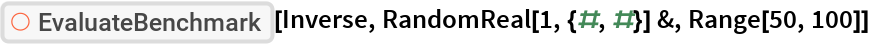Out=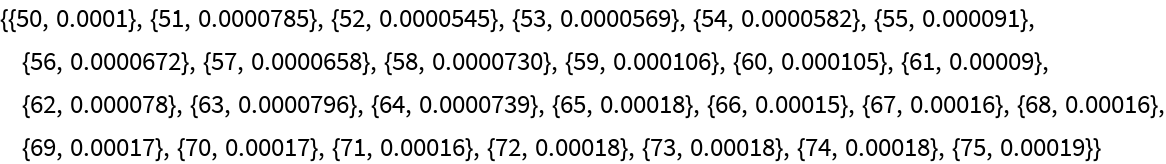View a plot of the results:

 In:=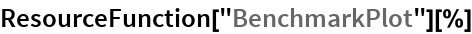Out=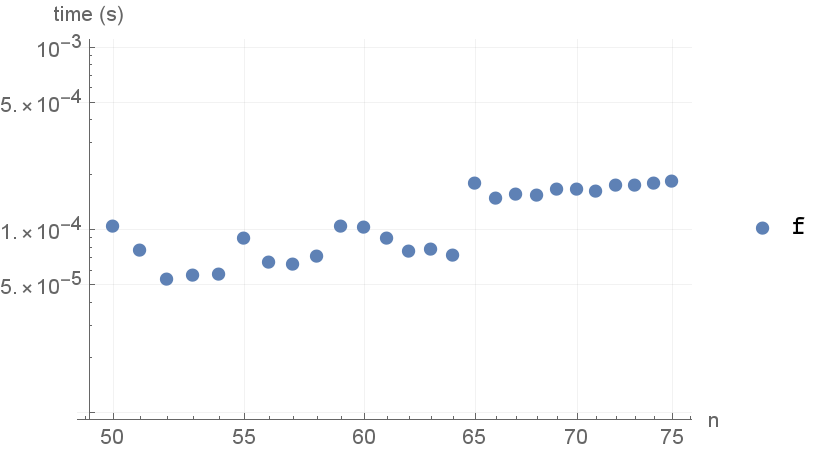### Options

#### TimeConstraint

By default, the total time is limited to 10 seconds:

 In:=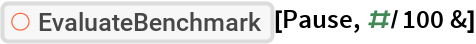Out=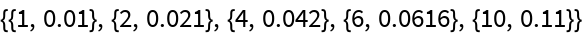Allow more time for benchmarking:

 In:=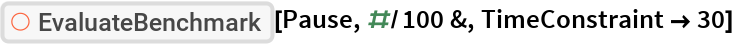Out=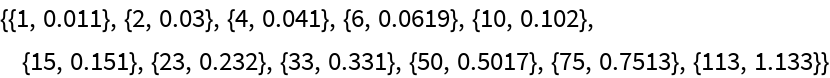Measure all inputs regardless of how long it takes:

 In:=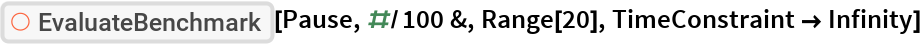Out=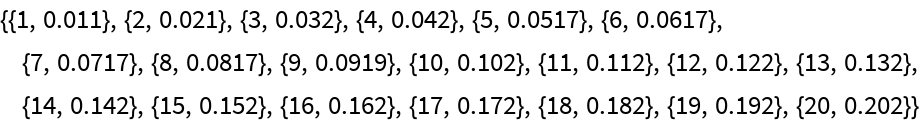In:=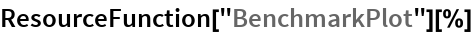Out=